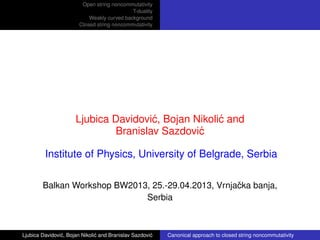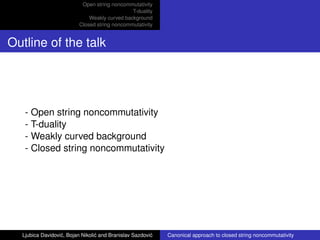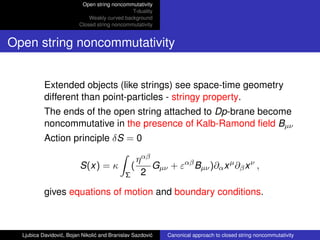Successfully reported this slideshow.

# B. Sazdović: Canonical Approach to Closed String Non-commutativity×

# B. Sazdović: Canonical Approach to Closed String Non-commutativity

Balkan Workshop BW2013
Beyond the Standard Models
25 – 29 April, 2013, Vrnjačka Banja, Serbia

Balkan Workshop BW2013
Beyond the Standard Models
25 – 29 April, 2013, Vrnjačka Banja, Serbia

### B. Sazdović: Canonical Approach to Closed String Non-commutativity

1. 1. Open string noncommutativity T-duality Weakly curved background Closed string noncommutativity Canonical approach to closed string noncommutativity Ljubica Davidovi´c, Bojan Nikoli´c and Branislav Sazdovi´c Institute of Physics, University of Belgrade, Serbia Balkan Workshop BW2013, 25.-29.04.2013, Vrnjaˇcka banja, Serbia Ljubica Davidovi´c, Bojan Nikoli´c and Branislav Sazdovi´c Canonical approach to closed string noncommutativity
2. 2. Open string noncommutativity T-duality Weakly curved background Closed string noncommutativity Outline of the talk - Open string noncommutativity - T-duality - Weakly curved background - Closed string noncommutativity Ljubica Davidovi´c, Bojan Nikoli´c and Branislav Sazdovi´c Canonical approach to closed string noncommutativity
3. 3. Open string noncommutativity T-duality Weakly curved background Closed string noncommutativity Open string noncommutativity Extended objects (like strings) see space-time geometry different than point-particles - stringy property. The ends of the open string attached to Dp-brane become noncommutative in the presence of Kalb-Ramond ﬁeld Bµν Action principle δS = 0 S(x) = κ ∫ Σ ( ηαβ 2 Gµν + εαβ Bµν)∂αxµ ∂βxν , gives equations of motion and boundary conditions. Ljubica Davidovi´c, Bojan Nikoli´c and Branislav Sazdovi´c Canonical approach to closed string noncommutativity
4. 4. Open string noncommutativity T-duality Weakly curved background Closed string noncommutativity Open string noncommutativity Solution of boundary conditions xµ = qµ − Θµν ∫ σ dσ1pν(σ1) , where qµ and pν are effective variables satisfying {qµ(σ), pν(¯σ)} = 2δµ νδs(σ, ¯σ). The coordinate xµ is the linear combination of effective coordinate qµ and effective momenta pν - source of noncommutativity. Pure stringy property {xµ (0), xν (0)} = −2Θµν , {xµ (π), xν (π)} = 2Θµν . Ljubica Davidovi´c, Bojan Nikoli´c and Branislav Sazdovi´c Canonical approach to closed string noncommutativity
5. 5. Open string noncommutativity T-duality Weakly curved background Closed string noncommutativity Open string noncommutativity Effective action S(q) = S(x)|bound.cond. = κ ∫ d2 ξ 1 2 GE µν∂+xµ ∂−xν , where GE µν = (G − 4BG−1 B)µν , Θµν = − 2 κ (G−1 E BG−1 )µν , are effective metric and noncommutativity parameter, respectively. Ljubica Davidovi´c, Bojan Nikoli´c and Branislav Sazdovi´c Canonical approach to closed string noncommutativity
6. 6. Open string noncommutativity T-duality Weakly curved background Closed string noncommutativity T-duality It relates string theories with different backgrounds. Compactiﬁcation on a circle has two consequences: momentum becomes quantized - p = n/R (n ∈ Z) , the new state arises (winding states N) x(π) − x(0) = 2πRN . Mass squared of any state M2 = n2 R + m2 R2 α′2 + oscillators , is invariant under n ↔ m and R ↔ ˜R ≡ α′/R . Ljubica Davidovi´c, Bojan Nikoli´c and Branislav Sazdovi´c Canonical approach to closed string noncommutativity
7. 7. Open string noncommutativity T-duality Weakly curved background Closed string noncommutativity T-duality Compactiﬁcation on circle of radius R is equivalent to compactiﬁcation on radius ˜R - purely stringy property. Dual action ⋆S has the same form as initial one but with different background ﬁelds ⋆ Gµν ∼ (G−1 E )µν , ⋆ Bµν ∼ Θµν , which are essentially parameters of open string noncommutativity. Ljubica Davidovi´c, Bojan Nikoli´c and Branislav Sazdovi´c Canonical approach to closed string noncommutativity
8. 8. Open string noncommutativity T-duality Weakly curved background Closed string noncommutativity T-duality Canonical T-duality transformations πµ ∼= κy′ µ , ⋆ πµ ∼= κx′µ . There is no closed string noncommutativity for constant Gµν and Bµν {πµ, πν} = 0 =⇒ {yµ, yν} = 0 . Ljubica Davidovi´c, Bojan Nikoli´c and Branislav Sazdovi´c Canonical approach to closed string noncommutativity
9. 9. Open string noncommutativity T-duality Weakly curved background Closed string noncommutativity Choice of background ﬁelds Gµν is constant and Bµν = bµν + 1 3Hµνρxρ ≡ bµν + hµν(x). bµν and Hµνρ are constant and Hµνρ is inﬁnitesimaly small. These background ﬁelds satisfy space-time equations of motion. Now Bµν is xµ dependent. Ljubica Davidovi´c, Bojan Nikoli´c and Branislav Sazdovi´c Canonical approach to closed string noncommutativity
10. 10. Open string noncommutativity T-duality Weakly curved background Closed string noncommutativity T-duality along all directions Generalized Buscher construction has two steps: gauging global symmetry δxµ = λµ which is a symmetry even Bµν is coordinate dependent ∂αxµ → Dαxµ = ∂αxµ + vµ α , xµ → ∆xµ inv = ∫ P dξα Dαxµ (this is a new step). Ljubica Davidovi´c, Bojan Nikoli´c and Branislav Sazdovi´c Canonical approach to closed string noncommutativity
11. 11. Open string noncommutativity T-duality Weakly curved background Closed string noncommutativity T-dual action and doubled geometry xµ → Vµ = −κΘµν 0 yν + (g−1 E )µν ˜yν [xµ → (yµ, ˜yµ)]. ⋆Gµν = (G−1 E )µν(∆V) and ⋆Bµν = κ 2 Θµν(∆V). T-dual action is of the form ⋆ S = κ2 2 ∫ d2 ξ∂+yµΘµν − (∆V)∂−yν , where Θµν ± (x) = − 2 κ ( G−1 E (x)Π±(x)G−1 )µν , Π±µν = Bµν(x)± 1 2 Gµν . Ljubica Davidovi´c, Bojan Nikoli´c and Branislav Sazdovi´c Canonical approach to closed string noncommutativity
12. 12. Open string noncommutativity T-duality Weakly curved background Closed string noncommutativity T-dual transformation laws ∂±xµ = −κΘµν ± (∆V)∂±yν ∓ 2κΘµν 0±β∓ ν (V) , ∂±yµ = −2Π∓µν(∆x)∂±xν ∓ β∓ µ (x) , where β± µ (x) = ∓ 1 6 Hµρσ∂∓xρ xσ . It is inﬁnitesimally small and bilinear in xµ. Expression for β± µ comes from the term ∫ d2 ξvµ +Bµν(δV)vν − = ∫ d2 ξβα µ (V)δvµ α . Ljubica Davidovi´c, Bojan Nikoli´c and Branislav Sazdovi´c Canonical approach to closed string noncommutativity
13. 13. Open string noncommutativity T-duality Weakly curved background Closed string noncommutativity Transformation laws in canonical form x′µ = 1 κ ⋆ πµ − κΘµν 0 β0 ν (V) − (g−1 E )µν β1 ν (V) y′ µ = 1 κ πµ − β0 µ(x) . These inﬁnitesimal βα µ -terms are improvements in comparison with ﬂat space. Also they are source of noncommutativity. Ljubica Davidovi´c, Bojan Nikoli´c and Branislav Sazdovi´c Canonical approach to closed string noncommutativity
14. 14. Open string noncommutativity T-duality Weakly curved background Closed string noncommutativity Choosing evolution parameter ∆xµ (σ, σ0) = xµ (ξ) − xµ (ξ0) = ∫ ( ˙xµ dτ + x′µ dσ) If we take evolution parameter orthogonal to ξ − ξ0 then we have ∆xµ (σ, σ0) = ∫ σ σ0 dσ1x′µ (σ1) , ∆yµ(σ, σ0) = ∫ σ σ0 dσ1y′ µ(σ1) . We use information from one background to compute Poisson brackets in T-dual one. Ljubica Davidovi´c, Bojan Nikoli´c and Branislav Sazdovi´c Canonical approach to closed string noncommutativity
15. 15. Open string noncommutativity T-duality Weakly curved background Closed string noncommutativity General structure, y {y′ µ(σ), y′ ν(¯σ)} = F′ µν[x(σ)]δ(σ − ¯σ) {∆yµ(σ, σ0), ∆yν(¯σ, ¯σ0)} = ∫ σ σ0 dσ1 ∫ ¯σ ¯σ0 dσ2F′ µν[x(σ1)]δ(σ1 − σ2) {yµ(σ), yν(¯σ)} = −[Fµν(σ) − Fµν(¯σ)]θ(σ − ¯σ) . Putting σ = 2π and ¯σ = 0, we get {yµ(2π), yν(0)} = −2π2 {⋆ Nµ, ⋆ Nν} = − Cρ Fµνρdxρ = −2πFµνρNρ , where Nµ is winding number, xµ(2π) − xµ(0) = 2πNµ, and Fµνρ = ∂Fµν ∂xρ are ﬂuxes. Ljubica Davidovi´c, Bojan Nikoli´c and Branislav Sazdovi´c Canonical approach to closed string noncommutativity
16. 16. Open string noncommutativity T-duality Weakly curved background Closed string noncommutativity General structure, x {x′µ (σ), x′ν (¯σ)} = F′µν [y(σ), ˜y(σ)]δ(σ − ¯σ) {∆xµ (σ, σ0), ∆xν (¯σ, ¯σ0)} = ∫ σ σ0 dσ1 ∫ ¯σ ¯σ0 dσ2F′µν [y(σ1), ˜y(σ1)]δ(σ1−σ2) {xµ (σ), xν (¯σ)} = −[Fµν (σ) − Fµν (¯σ)]θ(σ − ¯σ) . Putting σ = 2π and ¯σ = 0, we get {xµ (2π), xν (0)} = −2π2 {Nµ, Nν} = −2π(Fµνρ⋆ Nρ + ˜Fµνρ⋆ pρ ), where ⋆Nµ, and ⋆p are winding number and momenta for yµ yµ(σ = 2π) − yµ(σ = 0) = 2π⋆Nµ, yµ(τ = 2π) − yµ(τ = 0) = 2π⋆pµ Fµνρ = ∂Fµν ∂yρ , ˜Fµνρ = ∂Fµν ∂˜yρ are ﬂuxes. Ljubica Davidovi´c, Bojan Nikoli´c and Branislav Sazdovi´c Canonical approach to closed string noncommutativity
17. 17. Open string noncommutativity T-duality Weakly curved background Closed string noncommutativity Noncommutativity of y coordinates y Fµν(x) = 1 κ Hµνρxρ, Hµνρ is ﬁeld strength for Bµν. {yµ(2π), yν(0)} = −2π2 { ⋆ Nµ, ⋆ Nν} ∼= − 2π κ BµνρNρ . xµ(τ, σ) = xµ 0 + pµτ + Nµσ + osc. yµ(τ, σ) = y0µ + ⋆pµτ + ⋆Nµσ + osc. . Ljubica Davidovi´c, Bojan Nikoli´c and Branislav Sazdovi´c Canonical approach to closed string noncommutativity
18. 18. Open string noncommutativity T-duality Weakly curved background Closed string noncommutativity Nongeometric ﬂuxes ⋆Bµν depends on double space coordinates (yµ , ˜yµ) ⋆ Bµν = ⋆ bµν + Qµνρ yρ + ˜Qµνρ˜yρ . There are two ﬁeld strengths Rµνρ = Qµνρ + cycl. , ˜Rµνρ = ˜Qµνρ + cycl. . Rµνρ and ˜Rµνρ are nongeometric ﬂuxes. Ljubica Davidovi´c, Bojan Nikoli´c and Branislav Sazdovi´c Canonical approach to closed string noncommutativity
19. 19. Open string noncommutativity T-duality Weakly curved background Closed string noncommutativity Noncommutativity of x coordinates x Fµν (y, ˜y) = 1 κ [ Rµνρ yρ − (˜Rµνρ − 4 ˜Qµνρ )˜yρ ] {xµ (2π), xν (0)} = −2π2 {Nµ , Nν } = − 2π κ [ Rµνρ⋆ Nρ − (˜Rµνρ − 4 ˜Qµνρ )⋆ pρ ] , All Poisson brackets close on winding numbers Nµ, ⋆Nµ and momenta pµ, ⋆pµ. Coefﬁcients are: geometric ﬂux Hµνρ and nongeometric ﬂuxes Rµνρ and ˜Rµνρ. Ljubica Davidovi´c, Bojan Nikoli´c and Branislav Sazdovi´c Canonical approach to closed string noncommutativity
20. 20. Open string noncommutativity T-duality Weakly curved background Closed string noncommutativity Additional relations If we dualize all directions we have {xµ, yν} = 0. In the case of partial T-dualization xµ = (xi, xa) → (xi, ya, ˜ya) it holds {xi, ya} ̸= 0. Ljubica Davidovi´c, Bojan Nikoli´c and Branislav Sazdovi´c Canonical approach to closed string noncommutativity